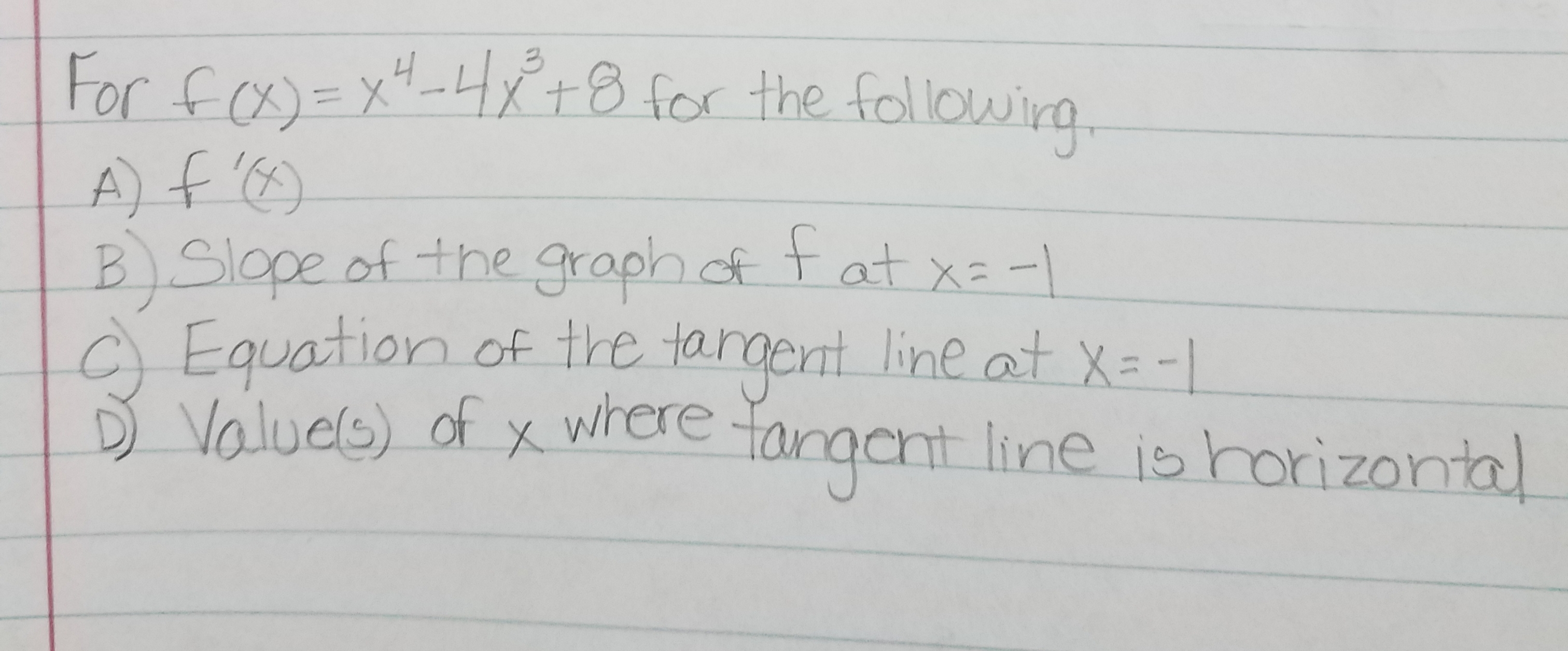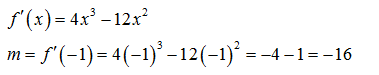For fx +8 for the followirgA)6)B.Slape of the graph ot tat xa-Equation of the targent line at X-Valuels) of x wrereaantne is horizonta

Questionhelp_outlineImage TranscriptioncloseFor fx +8 for the followirg A)6) B.Slape of the graph ot tat xa- Equation of the targent line at X- Valuels) of x wrere aant ne is horizonta fullscreen
Step 1

a)To find the derivative of f(x) , we use the result that derivative of x^n is n*x^(n-1)

Step 2

b)To find slope mof graph at x=-1 , we substitute x=-1 in f'(x)

Step 3

c)Now we find the value of y ...

Want to see the full answer?

See Solution

Want to see this answer and more?

Our solutions are written by experts, many with advanced degrees, and available 24/7

See Solution
Tagged in

Derivative